## Polyhedra

"Without Geometry life is pointless."
In "King of Infinite Space: Donald Coxeter, the Man Who Saved Geometry", by Siobhan Roberts.

The mathematician does not study pure mathematics because it is useful; he studies it because he delights in it and he delights in it because it is beautiful.
J. H. Poincaré

A polygon (plural "polygons") is a finite region of 2-D Euclidean space* bound by at least 3 straight line segments, or edges; these edges meet in pairs, in angles different from 0 and 180 degrees, at an identical number of vertices. These surface elements (henceforth "elements") - in this case edges and vertices - are, together with the inner surface, also part of the polygon.

A Regular polygon (henceforth Polygons) is isogonal and isotoxal. This means that not only are the elements identical (edges of the same length, edge-vertex-edge angles identical), but the polygon looks the same from all elements of a particular type. It follows from this that all elements of a particular type are equidistant from the centre of the polygon.

A Polyhedron (plural "polyhedra") is a finite region of 3-D Euclidean space** bound by at least 4 polygons - its "faces" - and at least 6 edges and 4 vertices. Just as its edges link two vertices, faces meet in pairs at its edges. Just as each polygonal face is bond by an equal number of edges and vertices (at least 3 each), edges and faces meet in equal numbers (and at least 3 each) at each vertex, in a way specified by the latter's polygonal "vertex figure". These elements are, together with the inner volume, also part of the polyhedron.

Regarding the paper models below, the conventions on polyhedral faces are as follows: The equilateral triangles (henceforth Triangles) are yellow, Squares are red, regular pentagons (Pentagons) are green and regular pentagrams (Pentagrams) are gold. The non-regular polygonal faces are blue.

* This is the traditional definition of a polygon. We will not consider here apeirogons, skew polygons, or complex polygons.

** Again, a traditional definition that excludes tesselations, which are infinite divisions of a 2-D Euclidean space - I don't have space in my office for infinite objects. However, polyhedra have a close analog in tesselations of 2-D spherical surfaces, the also finite spherical polyhedra.

### Regular and quasi-regular convex polyhedra

In a regular polyhedron, all faces and vertex figures are regular. From this, it follows that it is isohedral: the faces are not only identical, but the polyhedron looks the same seen from all of them; this implies, for instance, that all faces are equidistant from its centre. The same is true for the other types of elements, i.e., the polyhedron is also isogonal and isotoxal.

Regarding their names, we extend the polygonal naming convention to the regular polyhedra: For the regular tetrahedron, we will use simply the capitalized word Tetrahedron, and likewise for Octahedron, Icosahedron, Dodecahedron, etc.

The first models I built represented the five "Platonic solids", the convex regular polyhedra. They have been known at least since the time of the Pythagoreans.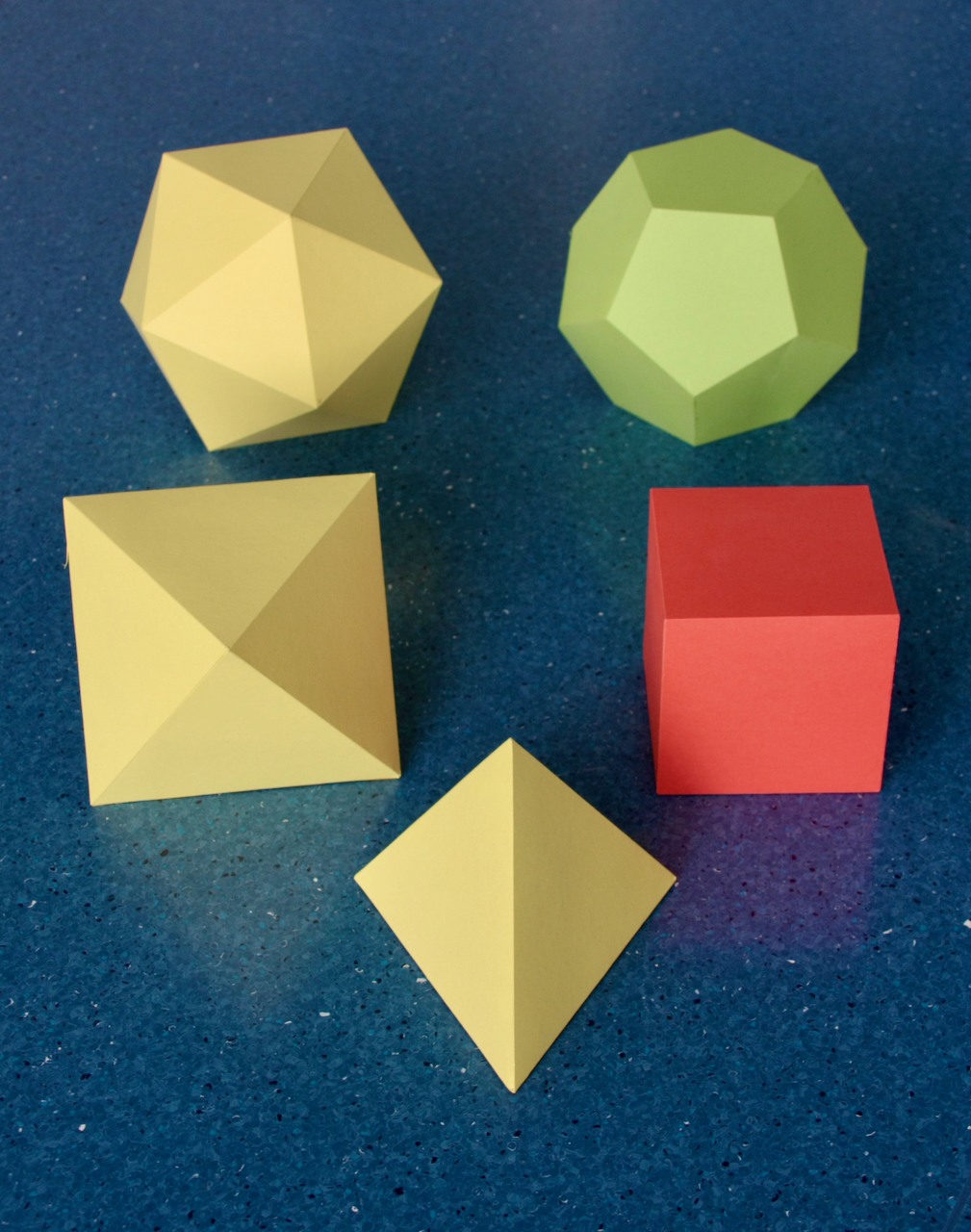Fig. 2a: The five Platonic solids.
In the foreground is the Tetrahedron.
In the middle row on the left is the Octahedron, to its right is the Cube.
In the background, on the left, is the Icosahedron, and on the right is the Dodecahedron.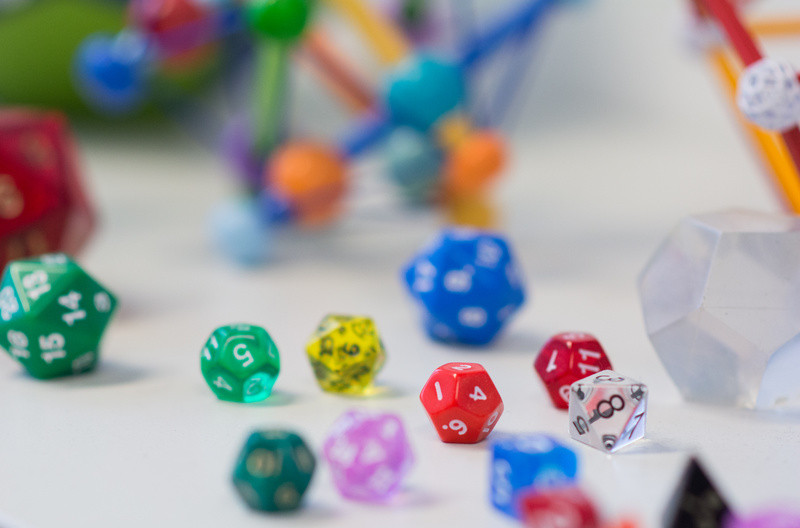Fig. 2b: Here are the platonic polyhedra again, this time as dice. Picture by Aris Noutsos.

The Tetrahedron has 4 Triangular faces, 4 vertices where 3 edges meet, i.e., with Triangular vertex figures, and 6 edges; it is an equilateral triangular pyramid. This is the smallest number of elements that any polyhedron can have, making this an analogue of the Triangle in 2 dimensions. It has tetrahedral symmetry.

The Octahedron has 8 Triangular faces, 6 vertices where 4 edges meet, i.e., with Square vertex figures, and 12 edges. One can think of it as two equilateral Square pyramids glued together at their Square bases. These Squares remain in the Octahedron as ``Equatorial'' polygons; there are three of them in three orthogonal planes. The Cube is the most familiar shape of all; it has 6 Square faces, 8 vertices with Triangular vertex figures and 12 edges. The Octahedron and the Cube have the same type of symmetry, called Octahedral symmetry.

The Icosahedron has 20 Triangular faces, 12 vertices with Pentagonal vertex figures and 30 edges. Note that its edges can highlight other polygons: the edges closer to each particular vertex that don't touch it trace a flat Pentagon. The Dodecahedron has 12 Pentagonal faces, 20 vertices with Triangular vertex figures and 30 edges. The Icosahedron and the Dodecahedron have the same type of symmetry, called Icosahedral symmetry.

That these are the only regular convex polyhedra can be proven by elementary geometry.

With the Platonic solids, we can build several interesting compounds. Two of them are presented below, the compound of an Octahedron and a Cube in Fig. 3a, and the compound of a Dodecahedron and an Icosahedron, see Fig. 3b. Note that their edges intersect in their midpoints, indicated by the black balls, at right angles.Fig. 3a: Zometool edge model of the compound of a Octahedron (edges in green) and a Cube (edges in blue).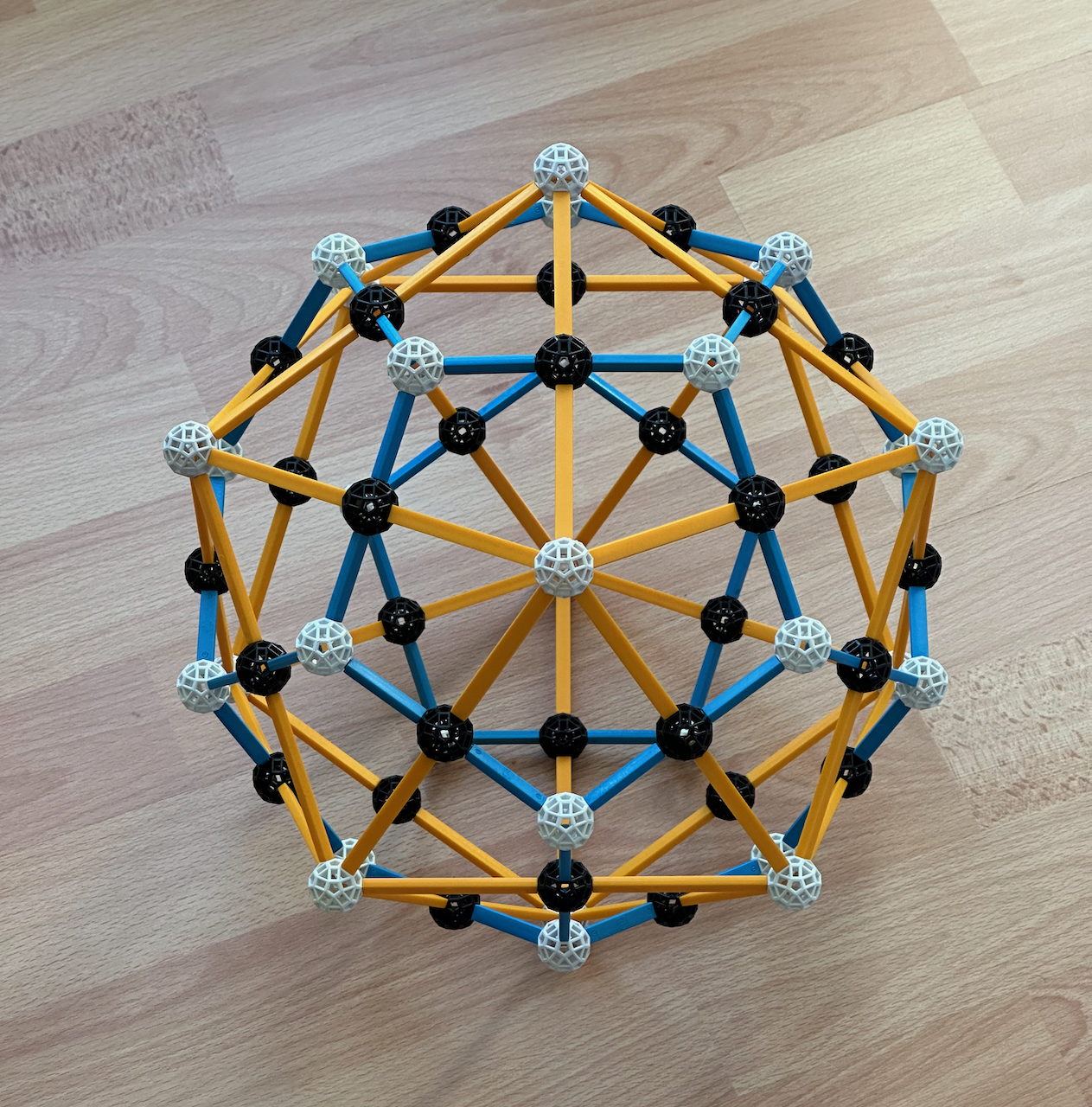Fig. 3b: Zometool edge model of the compound of a Icosahedron (edges in yellow) and a Dodecahedron (edges in blue).

The models in these figures were made for illustrative purposes only, they have been disassembled.

These compounds illustrate a concept that will be fundamental for understanding all polytopes, duality.

If two polygons A and B are dual, then to a vertex of A corresponds an edge of B, and to each vertex of B corresponds an edge of A. Thus, A and B are of the very same kind, but B is rotated relative to A by 180 deg / n, where n is the number of sides of the Polygons.

If two polyhedra A and B are dual, then to a vertex of A corresponds a face of B that is the dual polygon of the vertex figure of A, that face of B is in a plane perpendicular to the line from the vertex of A to its centre. Likewise, to a face of A corresponds a vertex of B, with a vertex figure that is the dual of the face of A. The number of edges of A and B are the same, and each edge of A intersects and edge of B at 90 degrees. If A and B are regular, their edges intersect in their midpoints.

As we can see from Fig. 3a, the Octahedron and the Cube are duals, in Fig. 3b, we can see that the Dodecahedron and Icosahedron are also duals. The dual of the Tetrahedron is another Tetrahedron in a different position (see Fig. 8a below), i.e., the Tetrahedron belongs to a special class of polyhedra that are self-dual.

***

In Fig. 4, we see models of the convex polyhedra derived from the (non-convex) polyhedron compounds in Figs. 3a and b: the convex hulls of the each compound, and the intersections of their constituent polyhedra.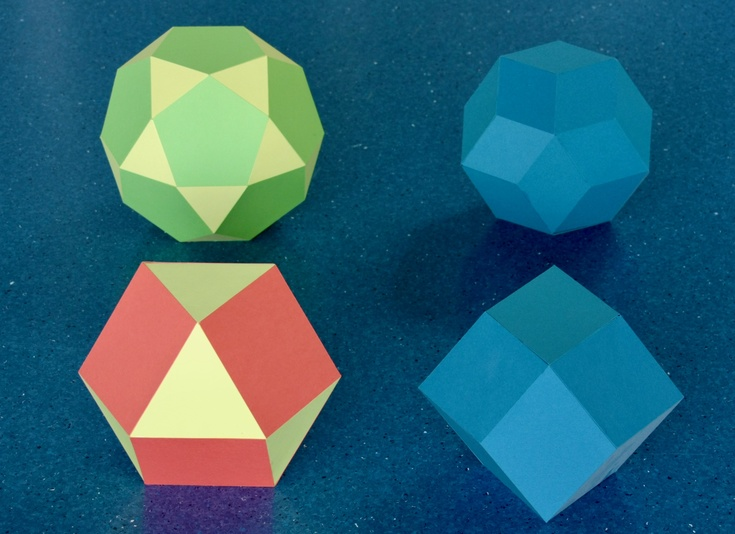Fig. 4: The convex quasi-regular polyhedra and the rhombic polyhedra.
Bottom left: the Cuboctahedron To its right is the Rhombic dodecahedron.
Top left: the Icosidodecahedron. To its right is the Rhombic triacontahedron.

The Cuboctahedron is the intersection of the two polyhedra in Fig. 3a. Its vertices are the black balls of that model. Given the way it is built, it has the 6 Square faces of the Cube, the 8 Triangular faces of the Octahedron, 12 vertices and 24 edges. The Rhombic dodecahedron is the convex hull of the two solids of the compound in Fig. 3a; its vertices are the full set of white balls of that compound. It has 12 Rhombic faces; hence its name. This has the 6 vertices of the Octahedron (with Square vertex figures) and the 8 vertices of the Cube (with Triangular vertex figures) and 24 edges. These polyhedra share the Octahedral symmetry of the compound from which they were derived.

The Icosidodecahedron is the intersection of the two solids in Fig. 3b, its vertices are the black balls of that model. It has the 20 triangular faces of the Icosahedron, the 12 pentagonal faces of the Dodecahedron, 30 vertices and 60 edges. The Rhombic triacontahedron is the convex hull of the compound in Fig. 3a. Its vertices are the full set of white balls in that model. Its has 30 Rhombic faces (hence its name), the 12 vertices of the Icosahedron (with Pentagonal vertex figures) and the 20 vertices of the Dodecahedron (with Triangular vertex figures), and also 60 edges. These polyhedra share the Icosahedral symmetry of the compound from which they were derived.

These polyhedra illustrate an important geometric operation called "rectification". It consists of marking the mid-points of the edges of a polyhedron (for instance, the black balls in Figs. 3a and b) and cutting off the vertices at those points. This results in a new face, provided those mid-points are co-planar.

The rectification of a regular polyhedron A, (rect(A)), has the following properties:
• Rect(A) has two types of faces. 1) The polygonal faces of A are replaced in rect(A) by their dual polygons (i.e., with that particular rotation); 2) Under the vertices of A, rect(A) has a new face, identical to the vertex figure of A.
• Since the vertices of rect(A) are the mid-points of the edges of A, their numbers are the same. The vertices of rect(A) are all identical, i.e., rect(A) is isogonal. Each vertex is touched by four edges and four faces, two faces of each type and alternating. Thus, the vertex figure is generally a rectangle, with its axes of symmetry aligned with the edges of A.
• The number of edges of rect(A) is twice the number of edges of A. All edges are identical and separate the two types of faces, i.e., rect(A) is isotoxal.
• If A and B are duals, Rect(A) = Rect(B). However, this is not true for dimensions higher than 3.
The rectification of the Octahedron and Cube is the Cuboctahedron in Fig. 4, the rectification of the Icosahedron and Dodecahedron is the Icosidodecahedron in Fig. 4. Importantly, if we rectify a Tetrahedron, the polyhedron we obtain has only one type of face (Triangles), which means it is regular: the Octahedron. The consequences of this will appear repeatedly in this and the pages that follow.

From this, it might seem that a rectification is the same as the intersection of two dual polyhedra whose edges intersect. That is the case for the rectifications of regular polyhedra, however, in general, the intersections of the edges of two dual polyhedra are not in their midpoints.

It is because they are isogonal and isotoxal that the Cuboctahedron and Icosidodecahedron (and only those rectifications) are called "quasi-regular". One of their characteristics is that they have "equatorial" polygons, a consequence of their rectangular vertex figures: this is a Square in the case of the Octahedron, a Hexagon in the case of the Cuboctahedron, and a Decagon in the case of the Icosidodecahedron (these equatorial polygons can be used with some of the previous faces to make Hemipolyhedra). These equatorial polyhedra makes it very easy to calculate their metric properties.

Now, each rhombic face of a rhombic polyhedron in Fig. 4, defined in Figs. 3a and b by the white balls, is centred on a black ball (a vertex of a quasi-regular polyhedron). Equally, we see in those Figures that each face of a quasi-regular polyhedron (defined by the black balls) is centred on a white ball. This means, as you might have guessed already, that the quasi-regular and rhombic polyhedra are duals.

Being the duals of the quasi-regular polyhedra, the rhombic polyhedra in Fig. 4 must have the following properties:
Since the quasi-regular polyhedra have equatorial polygons, the rhombic polyhedra have equatorial "rings" of rhombic faces.

One way of making a Rhombic dodecahedron is by dividing a Cube into six Square pyramids, and attach them by their Square bases to the faces of a second Cube. This operation can be seen as the dual of making a Cuboctahedron by rectification of the Octahedron. This will be important later for understanding the 24-cell, and why 4-dimensional space has so many regular polytopes. This also has an immediate consequence: if, in a filling of 3-D space with Cubes (the Cubic honeycomb) we select half of the Cubes in such a way that they touch each other only in their vertices, divide those into 6 Square pyramids and attach those pyramids to the neighbouring Cubes, we can fill the full 3-D space with Rhombic dodecahedra: this is the Rhombic dodecahedron honeycomb.

We could in principle apply other types of truncation operation to the Platonic solids to generate other polyhedra. There are, in total, 13 isogonal convex polyhedra where the faces, though not identical, are all Polygons: these are known as the Archimedean polyhedra. Apart from the Cuboctahedron and Icosidodecahedron, none of the other Archimedean polyhedra (or their duals, the Catalan solids) is isotoxal. So if you come to my office, don't ask me about the Truncated icosahedron: it is not even isotoxal!

### Star polyhedra

If we relax the condition of convexity, then there are four additional regular polyhedra, known as the Kepler-Poinsot polyhedra, or more simply the regular "star" polyhedra. These admit non-convex regular Polygons (Star polygons) as faces, however, only Pentagrams actually occur. The regular polyhedra with Pentagrammic faces were described in detail by Johannes Kepler. Their duals were found about 200 years later by Louis Poinsot. All of them have 30 edges and Icosahedral symmetry.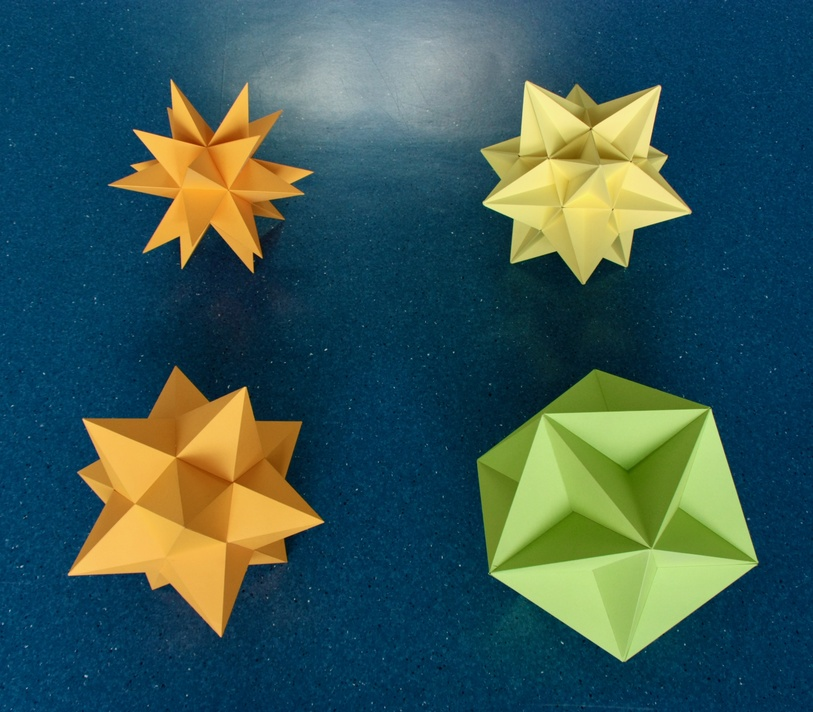Fig. 5: The four regular "star" polyhedra. Note how the central parts of their faces are hidden inside the models.
Bottom left: the Stellated dodecahedron. Bottom right: the Great dodecahedron
Top left: The Great stellated dodecahedron. Top right: the Great icosahedron.

The Small stellated dodecahedron (henceforth "Stellated dodecahedron" for short, see 3-D model here) has 12 Pentagrammic faces and 12 vertices with Pentagonal vertex figures. Its dual, the Great dodecahedron (see 3-D model here), has 12 Pentagonal faces (their edges can already be seen in the model of the Icosahedron) and 12 vertices with Pentagrammic vertex figures. The Great stellated dodecahedron (3-D model here) has 12 Pentagrammic faces and 20 vertices with Triangular vertex figures. Its dual, the Great icosahedron (3-D model here), has 20 Triangular faces and 12 vertices with Pentagrammic vertex figures.

As mentioned at the start, for regular polygons or polyhedra all vertices are at the same distance from the centre. For this reason, the inner vertices of the Pentagram or star polyhedra are "false vertices": edges intersect, but they don't end there. In what follows, we generally refer only to the true, outer vertices. Similarly for the edges of the non-convex polyhedra: two or more faces can intersect at a false edge, but none of those faces is limited by that edge. As an example, for the Great dodecahedron above, only the outer edges (with the same arrangement of the Icosahedron) are true edges.

We now introduce two very important concepts that are essential for understanding these polyhedra, stellation and faceting.

Among polygons, stellation means extending the edges of a polygon until they meet other similarly extended edges of the same polygon. Each stellation transforms a convex Polygon into a larger star polygon or a polygram, which is a regular compound of Polygons. Facetings cut into a polygon, but preserve its vertices. The larger the number of sides/vertices of a polygon, the larger the number of its possible stellations and facetings.

For polyhedra, things are similar: Stellations extend the polyhedron by extending its faces along their planes until they meet other similarly extended faces; therefore they preserve the number of faces and their facial planes. Facetings remove parts of a polyhedron while preserving its vertex arrangement. A very important rule links these operations: If polyhedron B is the dual of A, then the dual of a stellation of A, s(A) is a faceting of B, f(B). The reason is simple: if the facial planes of A and s(A) are the same, then the lines where we find the vertices of their duals, the perpendiculars to those planes, are also the same. Thus, B and the dual of s(A) have the same vertex arrangement, thus the latter is a faceting of B. This rule will be very important to undertand all that follows. The larger the number of faces/vertices of a polyhedron, the larger (in principle) is the possible number of its stellations/facetings.

The detailed geometric relations between the Dodecahedron, Icosahedron and the regular star polyhedra are displayed by the diagrams Ia and Ib below. All the operations depicted preserve the full Icosahedral symmetry. Some preliminary conclusions from these diagrams:
• The Stellated dodecahedron, Great dodecahedron, and Great stellated dodecahedron are regular stellations of the Dodecahedron, all have 12 faces as indicated by their names. The Great icosahedron, the only regular star polyhedron with 20 faces, is a regular stellation of the Icosahedron.
• Therefore, the duals of the three stellations of the Dodecahedron - the Great dodecahedron, Stellated Dodecahedron and Great Icosahedron - are all regular facetings of the Icosahedron, sharing its arrangement of 12 vertices. The dual of the Great icosahedron, the Great stellated dodecahedron, is the only regular star polyhedron with 20 vertices and a regular faceting of the Dodecahedron.
Following Coxeter (see references), in Diagram Ia we arrange these six polyhedra as the vertices of an imaginary regular Hexagon. This is the only Polygon where the distance of a vertex to the centre is the same as the length of the edge, i.e., it is radially equilateral. This vertex arrangement is, together with the centre, part of the optimal circle packing lattice.

The vertical scale indicates the density.
1. Duality. This operation is represented by the two-sided yellow arrows. Since this operation preserves the density, these arrows are horizontal.
2. Isomorphism. As pointed out by Coxeter, each line going through the centre of this Hexagon (here in red) changes a Pentagon into a Pentagram, or vice-versa, either in the faces or in the vertex figures of the polyhedra at the ends of the line. This results in isomorphic polyhedra. This means that the polyhedra at the ends of the red lines are topologically identical: they have the same number of faces, edges and vertices; the faces have the same number of sides, and the vertices have the same number of faces and edges converging into them, etc. (i.e., their configuration matrices are identical).

This hexagonal arrangement is useful for highlighting other geometric relationships between these polyhedra, which we represent in Diagram Ib by sets of parallel lines. There are two "stellation" operations that occur among regular polyhedra, these give the regular star polyhedra their names:
1. Edge stellation. This operation is represented as the blue arrows cutting through the Hexagonal arrangement, not through the edges or through the centre. It extends the edges of the faces (obviously in the same direction, but both ways) until they meet with other such extended edges. It stellates Pentagonal faces into Pentagrammic faces.
2. Greatening. This operation is represented by the green arrows. It replaces a face with a larger dual in the same plane (see Fig. 11a).
We also present the two faceting operations, which are the duals of the previous stellation operations.
1. Edge faceting. This operation, represented by the solid red lines is equivalent to: finding dual + edge stellating + finding dual. Since edge stellation preserves the edge directions, the dual operation preserves the perpendicular edge directions. Since this is a faceting, the vertex arrangement (the points where the edges end) are also preserved; this means that the whole edge arrangement is preserved under this transformation. Indeed, the Great dodecahedron has the same edge arrangement as the Icosahedron, and the Great icosahedron has the same edge arrangement of the Stellated dodecahedron, as can be seen in Fig. 5. The operation facets the pentagonal vertex figure into a Pentagram, a consequence of the fact that its dual operation, edge stellation, stellates Pentagonal faces into Pentagrams.
2. Vertex faceting. The faceting operation represented by the dashed lines is equivalent to: finding dual + greatening + finding dual. Since greatening transforms the faces into larger duals, this faceting operation replaces a vertex figure by a smaller dual.
The four polyhedra in the red circuit are facetings of the Icosahedron, sharing its vertex arrangement (see Fig. 11c). This is a necessary consequence of the fact that their duals are the stellations of the Dodecahedron (see Fig. 11b). The Great stellated dodecahedron is a faceting of the Dodecahedron (Fig. 12c); this is a necessary consequence of the fact that its dual, the Great icosahedron, is a stellation of the Icosahedron (Fig. 12b).

There are no other regular star polyhedra. All such polyhedra must be stellations and facetings of the Platonic solids because they must also be symmetric around each vertex and around each face. This means that the facial planes and vertices must have the same arrangements as those of one or two Platonic solids; there are no other symmetries (otherwise, there would be additional Platonic solids). If the facial plane and vertex arrangements are those of two different Platonic solids, then those must be dual to each other because they must have the same overall symmetry. These can only be the Icosahedron and/or Dodecahedron because, as we will see below, the only stellation of a simpler Platonic solid (of the Octahedron) and the only faceting of such a solid (of the Cube) are one and the same, the Compound of two tetrahedra.

***

We now rectify the four regular star polyhedra. From this, we obtain two quasi-regular star polyhedra, which like their convex kin are isotoxal, isogonal, have rectangular vertex figures and equatorial polygons. Their duals are therefore isotoxal and isohedral, with rhombic faces. All these operations preserve the Icosahedral symmetry of the regular star polyhedra.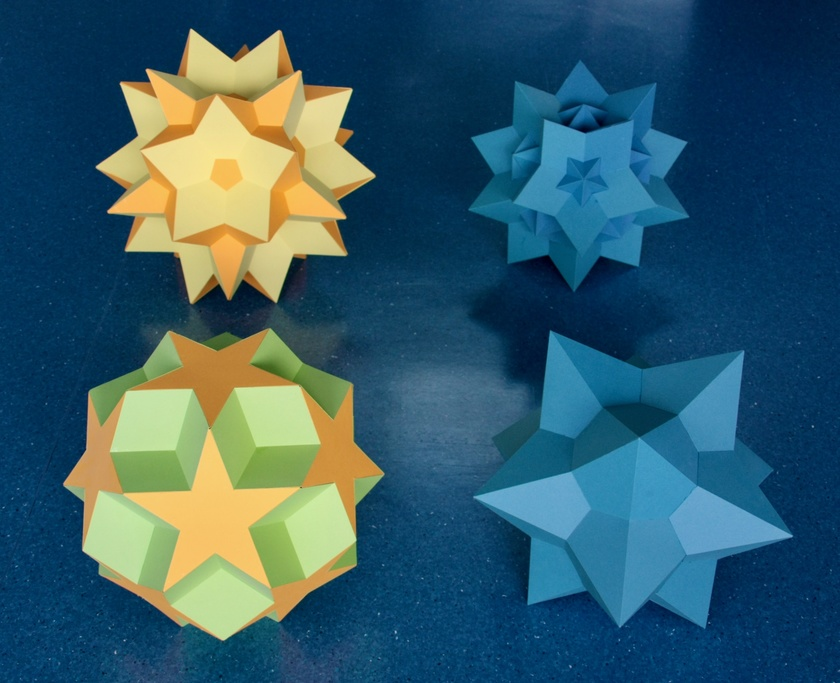Fig. 6a: Two quasi-regular star polyhedra and their duals.
Bottom left: the Dodecadodecahedron. Bottom right: Medial rhombic triacontahedron.
Top left: the Great icosidodecahedron. Top right: the Great rhombic triacontahedron.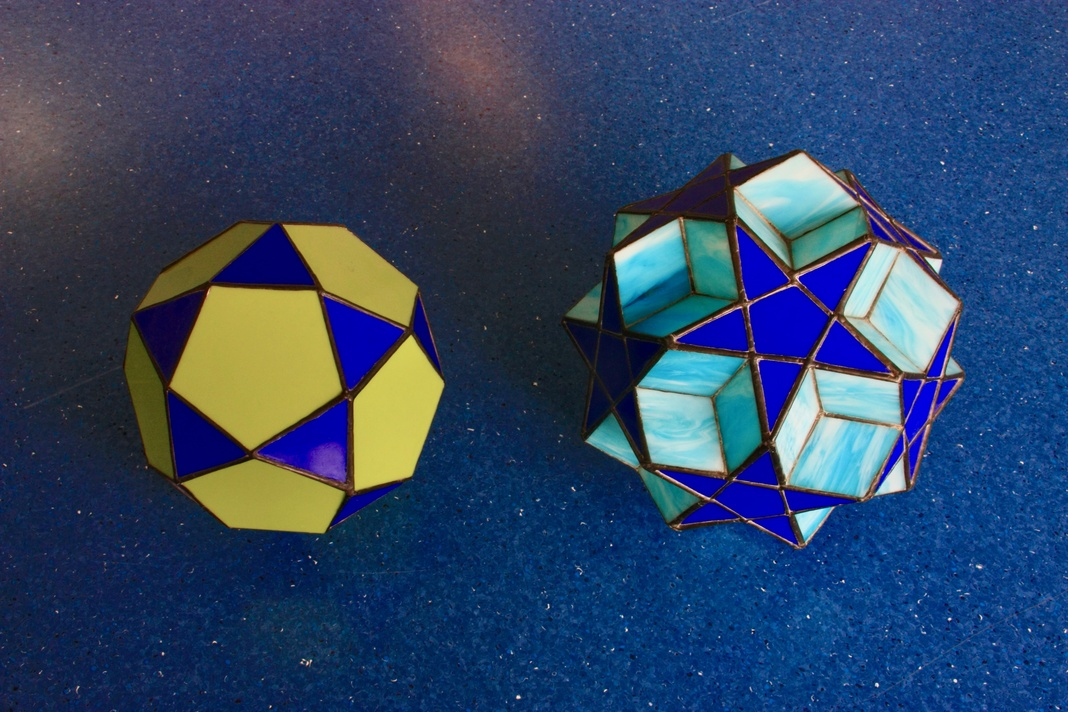Fig. 6b: Here are stained glass models of two quasi-regular polyhedra: the Icosidodecahedron (right) and the Dodecadodecahedron (left).

The Dodecadodecahedron is the rectification of the Great dodecahedron and the Stellated dodecahedron. It contains the 12 Pentagonal faces of the former and the 12 Pentagrammic faces of the latter. Its equatorial polygon is the Hexagon. Its dual is the Medial rhombic triacontahedron. The Great icosidodecahedron is the rectification of the Great icosahedron and the Great stellated dodecahedron. It contains the 20 triangular faces of the former and the 12 Pentagrammic faces of the latter. Its equatorial polygon is the Decagram. Its dual is the Great rhombic triacontahedron.

Being rectifications of the regular star polyhedra, the Dodecadodecahedron and the Great Icosidodecahedron must have 30 vertices (as many as the edges of the regular star polyhedra) with the vertex arrangement of the Icosidodecahedron. We can see this easily from Diagram Ib: the Stellated dodecahedron can be obtained from the Dodecahedron by a symmetric (both ways) edge stellation. Thus the arrangement of the mid-points of its 30 edges remained unchanged under edge stellation. i.e., it is still the vertex arrangement of the Icosidodecahedron. The same necessarily applies to its dual, the Great dodecahedron. The Great stellated dodecahedron can similarly be obtained from the Great dodecahedron by edge stellation, therefore the mid-points of its 30 edges still have the same arrangement as the Icosidodecahedron. The same necessarily applies to its dual, the Great icosahedron.

Since the rectifications of the regular star polyhedra are facetings of the Icosidodecahedron, their duals are necessarily stellations of the dual of the Icosidodecahedron, the Rhombic triacontahedron (see Figs. 14 a and b). All six polyhedra have 60 edges.

As we have seen, the Great icosahedron is topologically identical to the Icosahedron. For this reason, the Great icosidodecahedron, the rectification of the Great icosahedron, is topologically identical to the Icosidodecahedron, the rectification of the Icosahedron. The same applies, then, to their duals, the Rhombic triacontahedron and the Great rhombic triacontahedron.

***

We now show models of the ditrigonal polyhedra. They are not obtained by rectification of a regular polyhedron, they are instead special facetings of the Dodecahedron, which implies that their duals are stellations of the Icosahedron.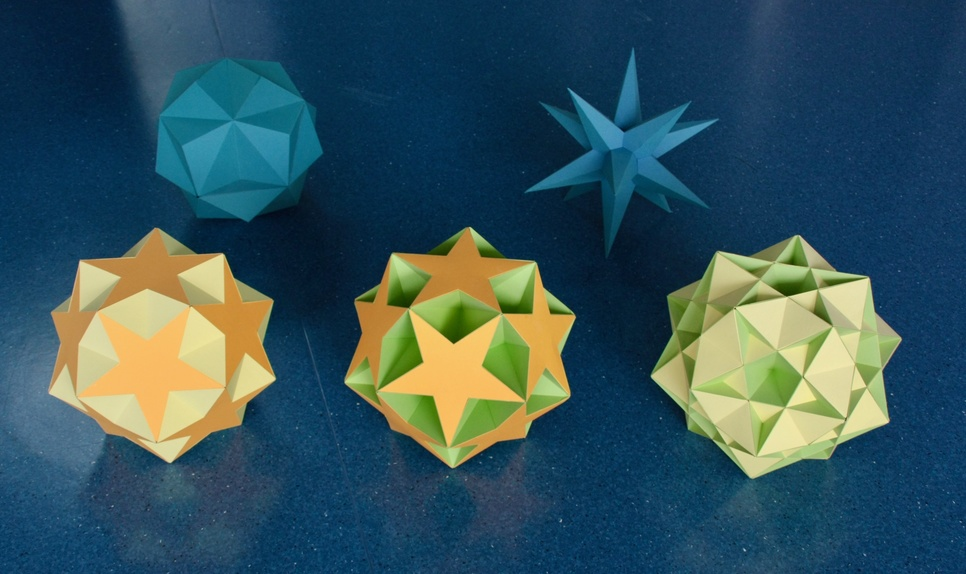Fig. 7: The ditrigonal polyhedra and their duals:
Bottom left: The Small ditrigonal icosidodecahedron; on top left is its dual, the Small triambic icosahedron.
Bottom middle: Ditrigonal dodecadodecahedron; on top right is its dual, the Medial triambic icosahedron.
Bottom right: The Great ditrigonal icosidodecahedron; on top right is its dual, the Great triambic icosahedron. This is represented by the same model as the Medial triambic icosahedron as they only differ in their internal structure.

Their properties are analogous to those of the rectifications above, this is why they are also classified as "quasi-regular": They also have two types of regular faces; in fact each ditrigonal polyhedron has the types and numbers of faces as one of the quasi-regular polyhedra with icosahedral symmetry! The Small ditrigonal icosidodecahedron has 12 Pentagrammic faces and 20 Triangular faces like the Great icosidodecahedron; the Ditrigonal dodecadodecahedron has 12 Pentagrammic and 12 Pentagonal faces like the Dodecadodecahedron, and the Great ditrigonal icosidodecahedron has 12 Pentagonal faces and 20 Triangular faces, like the Icosidodecahedron. All these polyhedra have 60 edges, all identical and separating the two types of faces. The ditrigonal polyhedra are also isogonal; the difference is that, instead of 4 faces and edges, they have 6 edges and 6 faces (three of each kind and alternating) converging on each vertex. Their vertex figures are therefore irregular hexagons with 3-fold symmetry. For this reason, they have no equatorial polygons.

Thus, their duals have two types of vertices - in the same numbers and with the same vertex figures as the vertices of the three Rhombic triacontahedra - they are isotoxal (with each of the 60 edges connecting the two types of vertices) and isohedral. The difference is that their faces, instead of rhombuses, are irregular hexagons with 3-fold symmetry, which are the duals of the hexagonal vertex figures of the dual ditrigonal polyhedra.

The three ditrigonal polyhedra share the same edge arrangement, which is also shared by the Compound of five cubes (see below). Therefore, the edges of all their duals (and thus the edges of the the dual of the Compound of five cubes, the Compound of five octahedra, see below) must all lie along the same set of perpendicular directions - indeed, they can all be derived from the first stellation of the Icosahedron (the Small triambic icosahedron) by a special type of asymmetric edge stellation.

Before ending this section, it is important to remark that the star polyhedra shown above represent only a small fraction of the uniform polyhedra.

### Regular and partially regular polyhedron compounds

When stellating or faceting a convex Polygon, we often obtain a compound Polygon, known as a Polygram. Likewise, stellating or faceting a polyhedron, the resulting figures are often polyhedral compounds.

Some of these are themselves fully regular. These must be stellations and facetings of the Platonic solids for the same reason as the regular star polyhedra: because they must also be symmetric around each vertex and around each face. The partially regular compounds are either stellations or facetings of a Platonic solid. Models of all of these are displayed next.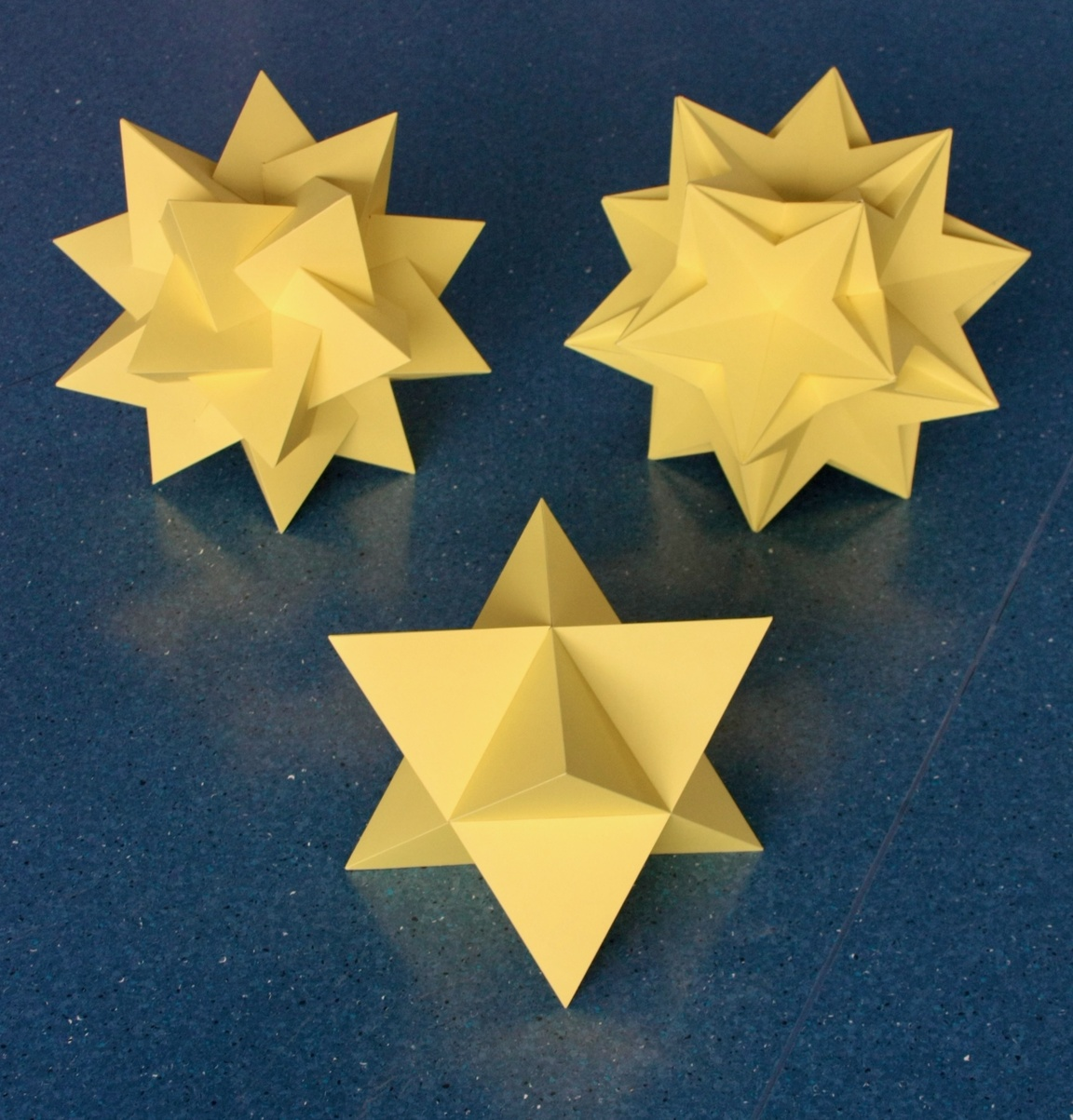Fig. 8a: The fully regular polyhedron compounds.
In the foreground: the Compound of two tetrahedra.
In the background, left: the Compound of five tetrahedra. On the right is the Compound of ten tetrahedra.

The Compound of two Tetrahedra is the only stellation of the Octahedron. The Triangular faces of the Octahedron are expanded into dual Triangles that are twice as large (see Figs. 10a, b). In Diagram Ib, this type of stellation is called a "greatening". The two Tetrahedra in the compound are dual to each other. Since the compound is self-dual, it is also a faceting of the Cube, the only one. It is also known as the "Stella octangula". This compound has Octahedral symmetry.

The Compound of five tetrahedra is a faceting of the Dodecahedron, but does does not share its Icosahedral symmetry; it only has rotational symmetry around the symmetry axes. This is known as Chiral Icosahedral symmetry. This means that this compound has a mirror image that is different from it, i.e., this compound comes in two Chiral forms. The existence of these forms implies that not all faceting and stellation operations preserve the symmetry of the polyhedra being faceted and stellated!

Interestingly, and very rarely, these forms are dual to each other. Since both are facetings of the Dodecahedron, then both of them must be stellations of the Icosahedron. Indeed, this compound has 20 Triangular faces, like the Icosahedron and Great icosahedron, and 20 vertices with Triangular vertex figures, like the Dodecahedron and Great stellated dodecahedron; like all regular polyhedra with Icosahedral symmetry, it has 30 edges.

The Compound of ten tetrahedra can be seen as the combination of the two chiral Compounds of five tetrahedra. Alternatively, it can be seen as a Compound of five Stellae octangulae, each made of Tetrahedra from the two dual Compounds of five tetrahedra. This compound is self-dual, and has full Icosahedral symmetry. It is also a faceting of the Dodecahedron and a stellation of the Icosahedron: The 40 vertices of the 10 Tetrahedra coincide in pairs with the 20 vertices of the Dodecahedron, and the 40 faces of the ten Tetrahedra fall in pairs on the 20 facial planes of the Icosahedron. This means that both Compounds of five tetrahedra facet the "same" Dodecahedron and stellate the "same" central Icosahedron. This compound has 60 edges.

As we said above, rectifying the Tetrahedron results in another regular solid, the Octahedron. Applying the process to the Compound of two Tetrahedra, we obtain two superposed Octahedra. An inspection of the model shows why this is the case: each new face produced by the rectification of a Tetrahedron is co-planar with a face of its dual Tetrahedron (see Figs. 10a ad b).

Applying the rectification to the other Tetrahedral compounds above, we obtain the results below.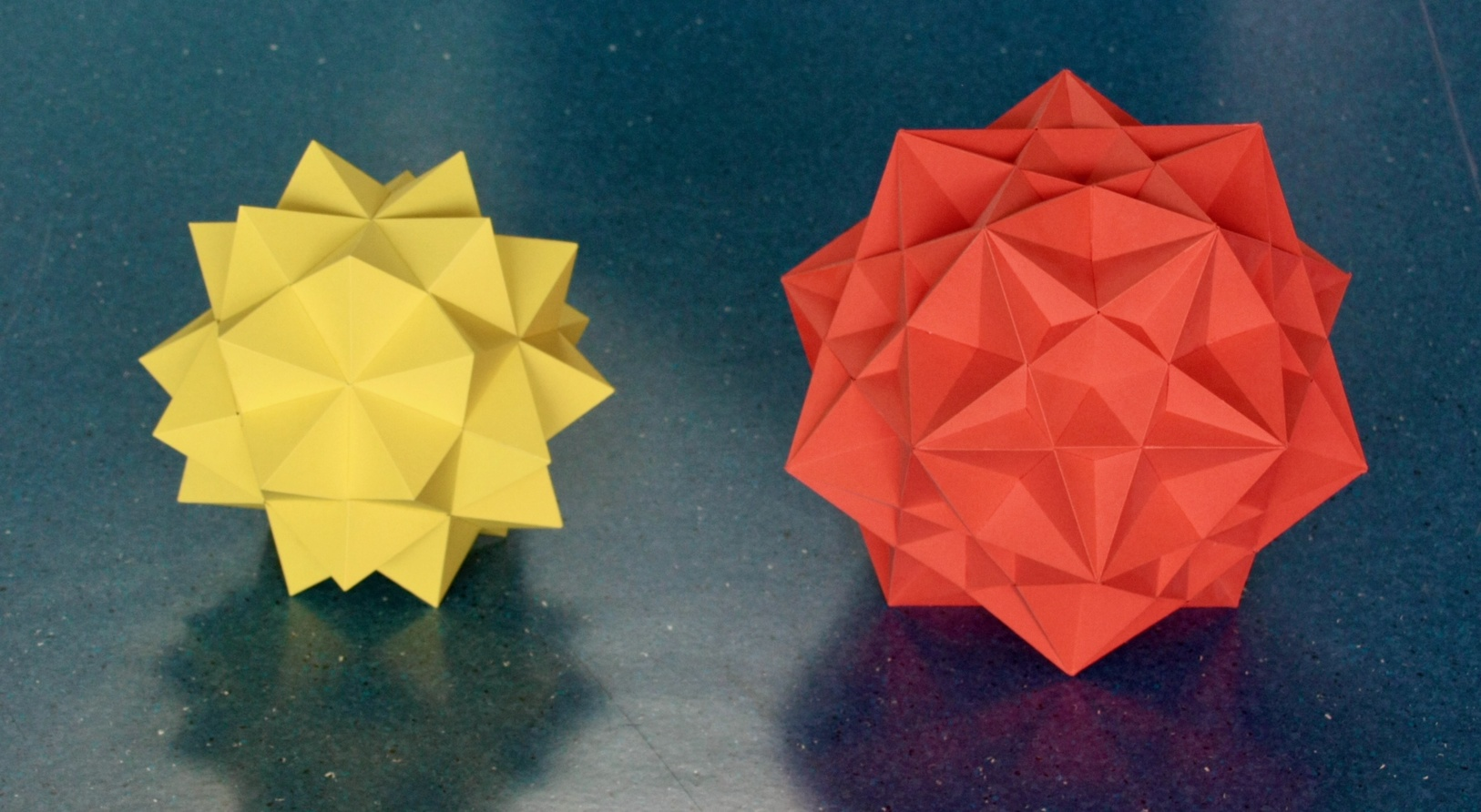Fig. 8b: The partially regular polyhedron compounds.
On the left is the Compound of five octahedra. On the right is its dual, the Compound of five cubes.

In the case of either Compound of five tetrahedra, the rectification results in a Compound of five octahedra. Rectifying the Compound of ten tetrahedra results in two exactly superposed Compounds of five octahedra; the reason is that the Compound of ten tetrahedra can be seen as a Compound of five Stellae octangulae, and as mentioned above, rectifying each of those results in two superposed Octahedra.
• Like the rectifications of regular star polyhedra in Fig. 6a, its 30 vertices (in this case of the 5 Octahedra) are arranged as the 30 vertices of the Icosidodecahedron.
• As above, the rectification doubles the number of edges of the Compound of five tetrahedra; this means that, like all quasi-regular polyhedra with Icosahedral symmetry and their duals, it has 60 identical edges. As for the duals of the ditrigonal polyhedra in Fig. 7, these 60 edges can be obtained by extending the of the first stellation of the Icosahedron, the Small triambic icosahedron.
• Like the duals to the ditrigonal polyhedra (Fig. 7), this is a stellation of the Icosahedron, this is why it is partially regular. The 40 Triangular faces of the 5 Octahedra fall in pairs on the 20 facial planes of the Icosahedron.
Indeed, the rectification of the Compound of five tetrahedra cuts its vertices in such a way that the new Triangular faces are also in the facial planes of a central Icosahedron (see detail in Fig. 9b). This happens because those new faces are co-planar with the faces of the dual Compound of five tetrahedra, which, as mentioned above, is also a stellation of the same Icosahedron.

Its dual, the Compound of five cubes, can alternatively be obtained by circumscribing each of the 5 Stellae octangulae of the Compound of ten tetrahedra with a Cube. It necessarily has the following properties:
• Like the duals of rectifications of regular star polyhedra (Fig. 6a), it is a stellation of the Rhombic triacontahedron. Indeed, the 30 faces of the 5 Cubes lie on the same planes as the 30 faces of the Rhombic triacontahedron (see Figs. 14a and b).
• It also has 60 identical edges. Because its dual is an edge stellation of the Small triambic icosahedron in Fig. 7, the Compound of five cubes is an edge-preserving faceting of the Small ditrigonal icosidodecahedron in Fig. 7.
• Like the ditrigonal polyhedra in Fig. 7, it is a faceting of the Dodecahedron, this is why it is also partially regular. The 40 vertices of its 5 Cubes coincide by two with the 20 vertices of the Dodecahedron.
Summarizing: Being facetings and stellations of the Platonic solids, the compounds in Fig. 8a are fully regular, like the regular star polyhedra in Fig. 5. Being rectifications of the compounds in Fig. 8a or their duals, the compounds in Fig. 8b share some characteristics with the rectified polyhedra in Fig. 6a; they are partially regular because they are edge facetings or edge stellations of the ditrigonal polyhedra and their duals in Fig. 7, which makes them facetings or stellations of the Platonic solids.

In practice, the two compounds in Fig. 8b are isogonal, isotoxal and isohedral, for that reason they are generally considered regular as well.

Among the regular star polyhedra, there were three regular stellations of the Dodecahedron and three regular facetings of the Icosahedron, but a single faceting of the Dodecahedron, and a single stellation of the Icosahedron. The regular and partially regular compounds add another 3 facetings of the Dodecahedron and 3 stellations of the Icosahedron. There are many more non-regular stellations of the Icosahedron: one counting method yields a total of 59 stellations (this includes the duals of the ditrigonal polyhedra in Fig. 7). It is not clear whether all of them have duals that are valid facetings of the Dodecahedron, but it is clear that some do, like the ditrigonal polyhedra in Fig. 7. Therefore, it is clear that the Dodecahedron admits many other non-regular facetings. In contrast, apart from the regular star polyhedra, there are no additional stellations of the Dodecahedron or facetings of the Icosahedron.

There are also other types of polyhedron compounds. One especially interesting class, which includes the objects we just discussed, is the uniform polyhedron compounds.

In what follows, we show some models that illustrate some of the geometrical relations between the objects we have just presented.

Main polytope page. Next: Polyhedra with Zometool